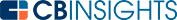# How to Calculate Compound InterestCompound interest (or “interest on interest”) is a powerful way to grow your money. Instead of traditional interest, where you earn interest just on an initial contribution, compound interest allows you to earn even more on the interest you’ve earned.

## What is compound interest?

Compound interest allows you to earn interest on the interest you earn from investing. Even without any additional deposits, compound interest allows your earnings to grow — as long as you don’t withdraw them. Compound interest only works if you let your funds sit in an account, untouched, for a length of time.

This principle can be used in either a positive way (in the form of investments and savings) or in a negative way (in the form of compounding debt). Ideally, compound interest helps you see more returns on money saved for retirement or other long-term investments.

Compound interest has a snowball effect on your money. It might take a while to get things going in the beginning, but with time, accounts with compounding interest grow faster the larger they are.

## How compound interest works

Here’s how compound interest works in practice:

• Create an account: You’ll open an account with a bank and deposit your principal. Make sure you sign up for an account that offers compounding interest!
• Start earning interest: Your bank will calculate interest on the original principal on a daily, monthly, or weekly basis. The more often your money compounds, the faster it grows the funds in your account. Be sure to choose an account that compounds more frequently to earn the most interest.
• Let it grow: Compounding interest can only work if you leave the principal amount in the account. Ideally, you would need to add even more funds to the account in addition to the principal, which would make it grow even faster. Over time, the account will accumulate value based on your principal and interest earned.

For example, say you deposit \$100 into an account that compounds at 5% annually. That means your account would earn \$5 a year in interest. After the first year, you have \$105 — your principal deposit plus interest earned.

The next year, you’ll earn an additional 5% on that \$105, which increases the balance to \$110.25. You might not get rich with a \$100 deposit. However, the higher your contributions, the interest rate, and the length of time, the more dramatically the value increases on a compounding account.

## How to calculate compound interest

It’s helpful to calculate compound interest so you can predict how long you’ll need to save, how much you’ll have for retirement, and more.

Here’s the official formula for calculating compound interest:

A = P (1 + [ r / n ]) ^ nt

Plug in these variables to understand how much compound interest your account will earn:

• A: This is what you’re calculating with the formula. This is the final value of your investment, plus interest.
• P: This is the initial deposit you put into the account, or the principal.
• R: This is the interest rate on an annual scale, added here as a decimal. For example, a 5% interest rate would be 0.05.
• N: How often your interest compounds. For example, if the interest compounds monthly (12 times in a year) or weekly (52 times a year).
• T: This stands for time. Plug in how long you’ll allow the money to compound.

Let’s say you have a savings account with these variables:

• P: You deposit \$1,000.
• R: You’re earning 5% interest on the money, or 0.05.
• N: The interest compounds monthly, 12 times a year.
• T: You’re allowing the money to compound for 15 years.

A = 1,000 (1 + [ 0.05 / 12 ]) ^ 12 X 15

This means you would earn \$2,113.70 over 15 years. The great thing about calculating compound interest is that you can adjust each variable as needed until you find the right mix of variables to get your desired results.

In a pinch, you can also use a reliable compound interest calculator to quickly calculate each variable

You may also like# www.cbinsights.com